# Texas Go Math Grade 5 Lesson 16.4 Answer Key Analyze Stem-and-Leaf Plots

Refer to our Texas Go Math Grade 5 Answer Key Pdf to score good marks in the exams. Test yourself by practicing the problems from Texas Go Math Grade 5 Lesson 16.4 Answer Key Analyze Stem-and-Leaf Plots.

## Texas Go Math Grade 5 Lesson 16.4 Answer Key Analyze Stem-and-Leaf Plots

A stem-and-leaf plot is useful when you need to see every item in a data set to solve a problem.

Examp1e 1.
The stem-and-leaf plot shows the points scored by hockey players this season. The average number of points scored last season was 35. How many players’ scores are greater than last year’s average? What are the scores?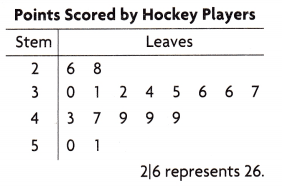• Locate 35 on the stem-and-leaf plot.
stem: ___ leaf: ____
• Count the numbers greater than 35.
There are ___ numbers greater than 35.
So, ___ players’ scores are greater than last year’s average.
The scores are _____

Math Talk
Describe another conclusion you can draw about the data in the stem-and-leaf plot.

• How many points did the greatest number of players score? Explain.

Example 2.
Solve a multi-step problem.
The stem-and-leaf plot shows the amounts of money the first 32 customers spent at the opening of an electronics store. Customers who spent $50 or more received a$12 coupon. Customers who spent less than $50 received a$7 coupon. What is the total value of the coupons the customers received?
Step 1
Find the number of customers who spent $50 or more. Multiply the number by$12.
____ × $12 =$_____
Step 2
Find the number of customers who spent less than $50. Multiply the number by$7.
____ × $7 =$____Step 3
Add to find the total value of the coupons.
$___ +$___ = $____ So, the total value of the coupon is$_____

Share and Show

Students made a stem-and-leaf plot to show daily high temperatures. Use the stem-and-leaf plot for 1-3.

Question 1.
What is the range of temperatures?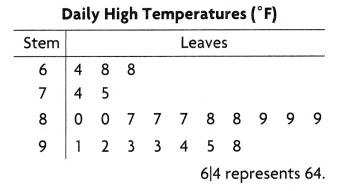5th Grade Go Math Answer Key for Stem and Leaf Plot Question 2.
On how many more days was the high temperature in the 80s than in the 90s?

Question 3.
What were the temperatures on the days when it was less than 80°F?

Problem Solving

Question 4.
H.O.T. What’s the Error? Jenny made this stem-and-leaf plot. She says that on the days when she did homework for less than 50 minutes, she spent a total of 5 hours doing homework. What’s the error?Question 5.
H.O.T. Multi-Step Pablo started to make a stem-and-leaf plot. He wrote the stems for the data: 1, 2, 3, 4, 5, and 6. He also wrote this key: 2|4 = 24. What is the least possible range of the data? What is the greatest possible range? Explain.

Problem Solving

The stem-and-leaf plot shows the number of text messages Chester received each day for 24 days. Use the stem-and-leaf plot for 6-7.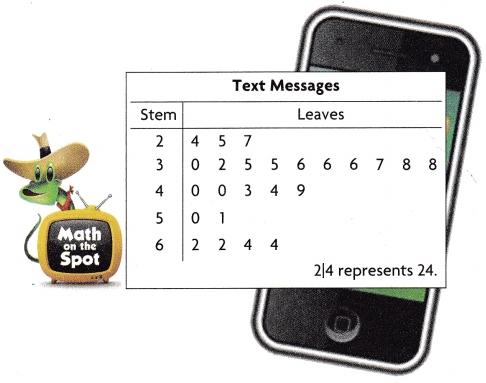Question 6.
H.O.T. Multi-Step On the days that Chester received more than 50 text messages, he responded to only – of the messages. How many text messages did Chester respond to?

Stem and Leaf Plot Answer Key 5th Grade Go Math Question 7.
Wite Math Explain how you can use the stem-and-leaf plot to make a reasonable prediction about the number of text messages Chester will receive each day next week.

Use the stem-and-leaf plot for 8-10.

Question 8.
Tom asked people in a survey how much money they could find under their sofa cushions. The stem-and-leaf plot shows the amounts of money. What is the least amount of money a person said they could find?
(A) 4¢
(B) 45¢
(C) 46¢
(D) 456¢

Question 9.
Which statement about the data is true?
(A) Two people each found 70¢.
(B) Two people each found 7¢.
(C) One person found 56¢.
(D) Eight people found 37¢.

Question 10.
Multi-Step Suppose Torn gives two concert tickets to everyone who participates in the sofa cushion survey. How many tickets does he give away?
(A) 10 tickets
(B) 14 tickets
(C) 28 tickets
(D) 38 tickets

Texas Test Prep

How many people live less than 40 miles from work?(A) 4
(B) 10
(C) 9
(D) 11

### Texas Go Math Grade 5 Lesson 16.4 Homework and Practice Answer Key

Students made a stem-and-leaf plot to show daily low temperatures. Use the stem-and-leaf plot for 1-3.

Question 1.
What is the range of temperatures? Write an equation to show how you found your answer,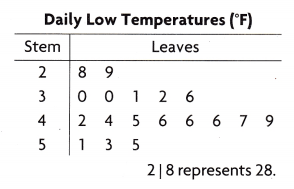Question 2.
On how many days was the low temperature in the 20s or the 30s?

Question 3.
What were the temperatures on the days when the low temperature was greater than 45°F?

Problem Solving

Alexandra posted a video on the Internet of her dog performing a trick. The stem-and-leaf plot shows the number of times Alexandra’s video was viewed each minute during a 20-minute period. Use the stem-and-leaf plot for 4-5.

The average number of views per minute for a video on a pet owner’s website is 60. How many times was the number of views of Alexandra’s video less than the average? _____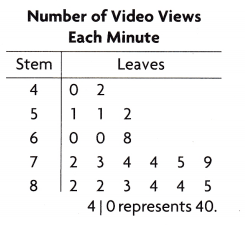Question 5.
Alexandra collects data for the next 5 minutes and finds that the number of views each minute are 88, 86, 95, 87, and 99. Explain how you can use the stem-and-leaf plot to make a reasonable prediction about the popularity of her video.

Lesson Check

Texas Test Prep

Question 6.
How many scores are greater than 20?Question 7.
Multi-Step Half the number of guesses are less than what number?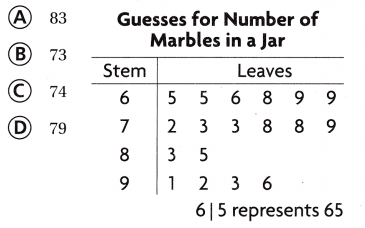The stem-and-leaf plot shows the number of carnival tickets sold each day. Use the stem-and-leaf plot for 8-11.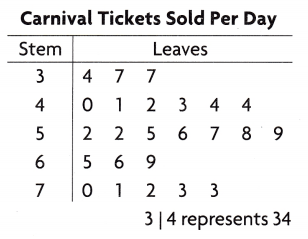Stem and Leaf Plot Worksheet with Answers Pdf Question 8.
Ticket sales for the next two days are 55 tickets and 68 tickets. Which statement about the data is true?
(A) The range stays the same.
(B) The range increases by two.
(C) The range decreases.
(D) The range doubles.

Question 9.
How many days were carnival tickets sold?
(A) 24 days
(B) 29 days
(C) 20 days
(D) 39 days

Question 10.
Multi-Step On any day that the number of tickets sold is greater than 70, twice that number of tickets is donated to a children’s hospital. How many tickets are donated to the hospital?
(A) 289
(B) 718
(C) 432
(D) 578